# What Is A Geometric Sequence? (10 Common Questions Answered)

Geometric sequences are used in mathematics whenever we have a sequence of numbers that grows or shrinks by a fixed percentage at each step.  However, there are a few things you should know about these sequences.

So, what is a geometric sequence?  A geometric sequence is a sequence of numbers that increases or decreases by the same percentage at each step. The ratio between consecutive terms in a geometric sequence is always the same. This ratio r is called the common ratio, and the nth term of a geometric sequence is given by an = arn.

Of course, a geometric sequence can have positive or negative terms.  The common ratio r can also be positive or negative.

Let’s get started.

## What Is A Geometric Sequence?

A geometric sequence (or geometric progression) is a sequence of numbers that increases or decreases by the same percentage at each step.  That is, the ratio between two consecutive terms in a geometric sequence is always the same.

This ratio, r, is called the common ratio of the geometric sequence.  If the nth term of a geometric sequence is an, then the common ratio r is:

• r = an+1 / an

Note that this formula also tells us how to find the next term in the sequence from the previous term.  If we solve for an+1, we get:

• an+1 = anr

We can use any nonnegative value of n to find the value of r.  For example, if we choose n = 0, then we only need the first two terms of the geometric sequence to find r:

• r = a1 / a0

Note that according to this convention, the term with index n = 0 is the first term – we see this often in computer science as well.

If we choose n = 10, we would need the 10th and 11th terms of the geometric sequence to find r:

• r = a11 / a10

### How To Find The Common Ratio Of A Geometric Sequence

If we have two consecutive terms in a geometric sequence, we can simply take their ratio (quotient) to find the common ratio r (as we did in the examples above).  Just remember to divide in the correct order, since division is not commutative.

For example, given a1 = 8 and a2 = 32, we would calculate:

• d = a2 / a1
• =32 / 8
• =4

The common ratio for this geometric sequence would be r = 4.  It would be incorrect to calculate 8 / 32 = 1 / 4.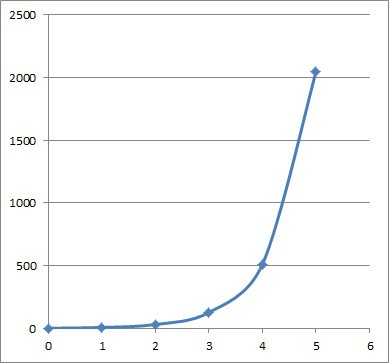The first several numbers in the geometric sequence with first term 2 and common ratio 4. Note that the curve displays exponential growth.

In general, the term with the larger index (the number in the subscript) comes first (it is the dividend or numerator), and the term with the smaller index is the divisor or denominator.

If we have two terms that are not consecutive, we need to divide them (again, in the proper order) and then take a root (the correct root is given by the difference between the larger index and the smaller index).

So, given two terms am and a in a geometric sequence (with m < n), we would find the common ratio r with the formula:

• r = (an / am)1/(n-m)

For example, given the two terms a3 = 8 and a12 = 4096 in a geometric sequence, we would use the formula above to calculate d with m = 3, n = 12, am = 8, and an = 4096:

• r = (an – am) / (n – m)
• r = (a12 / a3)1/(12-3)
• r = (4096 / 8)1/9
• r = (512)1/9
• r = 2

So, the common ratio for this geometric sequence is r = 2.  With this information, we can work backwards to find the first term a0 if we want to:

• a3 = 8
• a2 = 4  [since a3 = a2r, with a3 = 8 and r = 2]
• a1 = 2  [since a2 = a1r, with a2 = 4 and r = 2]
• a0 = 1  [since a1 = a0r, with a1 = 2 and r = 2]

We can find a general formula for the first term a0 by solving the equation from before with m = 0:

• r = (an / am)1/(n-m)
• r = (an / a0)1/(n-0)  [use a = 0]
• r = (an / a0)1/n
• r = (an / a0)1/n
• rn = an / a0  [raise both sides to the power of n]
• rn / an = 1 / a0  [divide both sides by an]
• an / rn = a0  [take the reciprocal of both sides]

So, given the nth term an and the common ratio r of a geometric sequence, we can find the first term a0 by the equation a0 = an / rn.

#### Does A Geometric Sequence Always Increase?

A geometric sequence does not always increase.  For example, if the first term a0 is positive and the common ratio r is positive and less than 1, the geometric sequence will decrease.

Take a0 = 8 and r = 1/4.  Then the first several terms of the sequence are 8, 2, 1/2, 1/8, 1/32, … which are decreasing terms.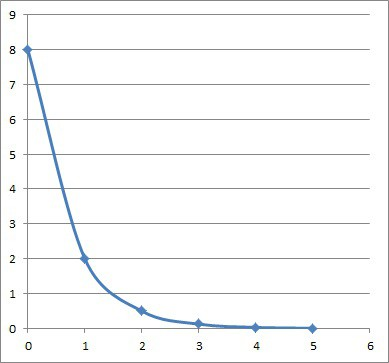The first several numbers in the geometric sequence with first term 8 and common ratio 1/4. Note that the curve displays exponential decay.

Keep in mind that a geometric sequence may not always increase or decrease.  A geometric sequence can also oscillate between positive and negative values.

If the common ratio r is negative, then the terms of the geometric sequence will switch between positive and negative.

Take a0 = 1 and r = -2.  The first several terms of the sequence are 1, -2, 4, -8, 16, -32, 64, … which switch between positive and negative.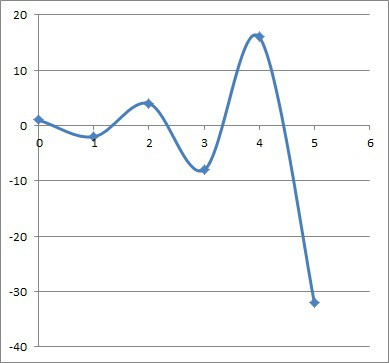The first several numbers in the geometric sequence with first term 1 and common ratio -2. Note that the points oscillate between positive and negative values, increasing in absolute value.

#### Does Every Geometric Sequence Converge?

A geometric sequence does not always converge. A geometric sequence converges if |r| < 1 (that is, -1 < r < 1).

The reason is that as the index n increases, the nth term an approaches zero (since rn approaches zero when |r| < 1).

If r = 1, the geometric sequence converges to a0 (a ratio of r = 1 means all the terms have the same value of a0 and the sequence converges to a0).  The terms would be a0, a0, a0, a0, …

If r = -1, the geometric sequence oscillates between a0 and –a0.  The terms would be a0, -a0, a0, -a0, …

If |r| > 1, the geometric sequence does not converge, since the terms will get larger in absolute value (get further away from zero) as n increases.

### How To Find The Nth Term Of A Geometric Sequence

To find a general formula for the nth term of a geometric sequence, we need the first term a0 and the common ratio r.

We can use the equation for a0 we found above and solve for an instead of a0:

• an / rn = a0  [equation from above]
• an = a0rn  [multiply by rn on both sides]

So, given the 1st term a0 and the common ratio r of a geometric sequence, we can find the nth term an by the equation an = a0rn.

#### Example: How To Find The Nth Term Of A Geometric Sequence

Let’s say we are given the terms a5 = 486 and a8 = 13,122 of a geometric sequence.  First, we will find r by the equation from earlier:

• r = (an / am)1/(n-m)
• r = (13,122 / 486)1/(8-5)
• r = (27)1/3
• r = 3

Now we need to find a0.  We will use the formula for a0 that we found earlier, along with a5 = 486:

• a0 = an / rn
• a0 = a5 / r5  [use n = 5]
• a0 = 486 / 35  [a5 = 486 and r = 3]
• a0 = 486 / 243
• a0 = 2

Now we can find the formula for the nth term an by the equation from earlier:

• an = a0rn
• an = 2*3n  [a0 = 2, r = 3]

We can check this formula for the 3 terms we already have to confirm:

• a0 = 2*30 = 2*1 = 2 (correct!)
• a5 = 2*35 = 2*243 = 486 (correct!)
• a8 = 2*38 = 2*6,561 = 13,122 (correct!)

So, the formula for the nth term of this geometric sequence is an = 2*3n.

The first several terms of the geometric sequence are 2, 6, 18, 54, 162, 486, 1,458, …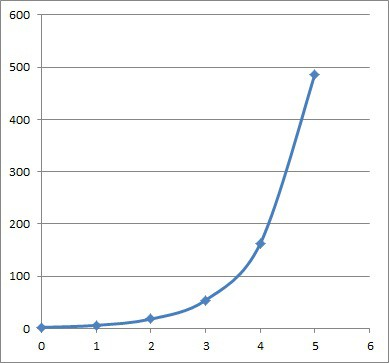The first several numbers in the geometric sequence with first term 2 and common ratio 3. Note that the curve displays exponential growth.

### What Is The Recursive Formula For A Geometric Sequence?

The recursive formula for a geometric sequence is the one involving the next term, the previous term, and the common ratio r (the same one we saw earlier):

• an+1 = anr

It is called recursive because it refers to a previous term to find the next term.

### Can Geometric Sequences Be Negative?

Geometric sequences can have negative terms.  In fact, every term of a geometric sequence will be negative if:

• the first term a0 is negative
• the common ratio r is positive

Of course, a geometric sequence may have some terms or no terms negative.

For example, if the common ratio r is negative, then every other term will be negative (and every other term will be positive).

If the first term a0 is positive and the common ratio r is positive, then all terms will be positive.

#### Example of A Geometric Sequence That Is Negative (All Terms Negative)

Consider the geometric sequence with first term a1 = -5 and common ratio d = 2.   All of the terms in this sequence will be negative, since:

• the first term a0is negative
• the common ratio r is positive

We can prove this with the general formula for the nth term of a geometric sequence:

• an = a0rn  [formula for nth term of any geometric sequence]
• an = (-5)*2n  [a0 = -5, r = 2]
• an = -(5*2n)

Since 5*2n is positive for any natural number n, we know that an = -(5*2n) will be negative for any natural number n.  Thus, every term of this geometric sequence will be negative.

The first several terms will be -5, -10, -20, -40, -80, -160, -320, -640, -1280, …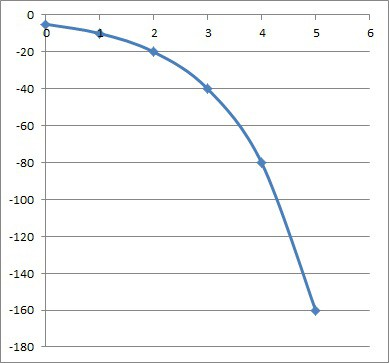The first several numbers in the geometric sequence with first term -5 and common ratio 2. Note that the curve displays exponential growth, but in a negative direction.

### Can Geometric Sequences Have Decimals Or Fractions?

Geometric sequences can have decimals or fractions.  This can happen if either:

• the first term a0 involves decimals or fractions (a0 is not a whole number)
• the common ratio r involves decimals or fractions (r is not a whole number)

#### Example of A Geometric Sequence With Decimals

Consider the geometric sequence with first term a0 = 4 and common ratio r = 1/2.   All but the first 3 terms in this sequence will have decimals, since:

• the common ratio r is not a whole number

The first several terms will be 4, 2, 1, 1/2, 1/4, 1/8, 1/16, 1/32, 1/64, 1/128, …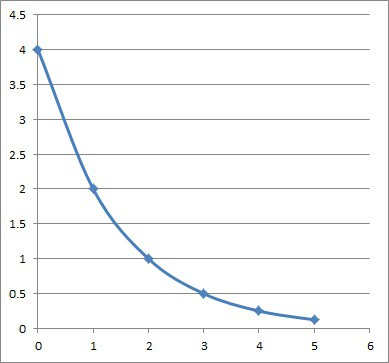The first several numbers in the geometric sequence with first term 4 and common ratio 1/2. Note that the curve displays exponential decay.

### Is Compound Interest A Geometric Sequence?

You can think of compound interest as a geometric sequence.  If the interest rate is Rint, then the common ratio is r = 1 + Rint.

For example, with an annual compound interest rate of 5% (Rint = 0.05), we have a geometric sequence with a common ratio of r = 1 + Rint = 1 + 0.05 = 1.05.

If we start with \$10,000 (a0 = 10,000), we can calculate the balance after n years with the equation:

• an = a0rn [general formula for a geometric sequence]
• an = 10,000*(1.05)n [with a0 = \$10,000 and r = 1.05]

For example, we can find:

• a1 = 10,000*(1.05)1 = \$10,500  [the account balance after 1 year]
• a2 = 10,000*(1.05)2 = \$11,025  [the account balance after 2 years]
• a3 = 10,000*(1.05)3 = \$11,576.25  [the account balance after 3 years]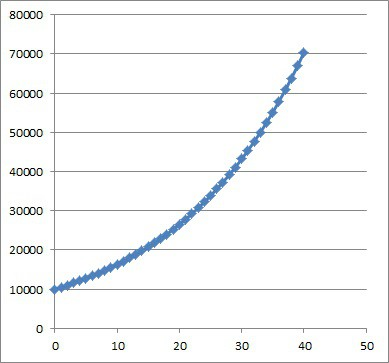This graph shows the first 40 years of compound interest at 5% for a starting balance of \$10,000. Note that the curve displays exponential growth.

Note that simple interest is an arithmetic sequence.

### Is The Fibonacci Sequence A Geometric Sequence?

The Fibonacci Sequence is not a geometric sequence, since it does not have the proper form: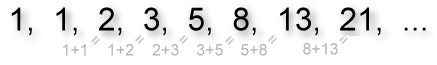Each term in the Fibonacci sequence is defined as the sum of the previous two terms (the first two terms are both taken to be one).

To find the nth term of the Fibonacci sequence, we use the formula:

• an = an – 1 + an – 2

where a1 = 1 and a2 = 1.

Remember the key difference:

• Geometric Sequence: to get the next term, take the previous term and multiply by a constant value (the common ratio r, which never changes)
• Fibonacci Sequence: to get the next term, take the previous term and add the 2nd previous term (the terms vary, and there is no common ratio between consecutive terms).

The first several terms of the Fibonacci sequence are 1, 1, 2, 3, 5, 8, 13, 21, 34, …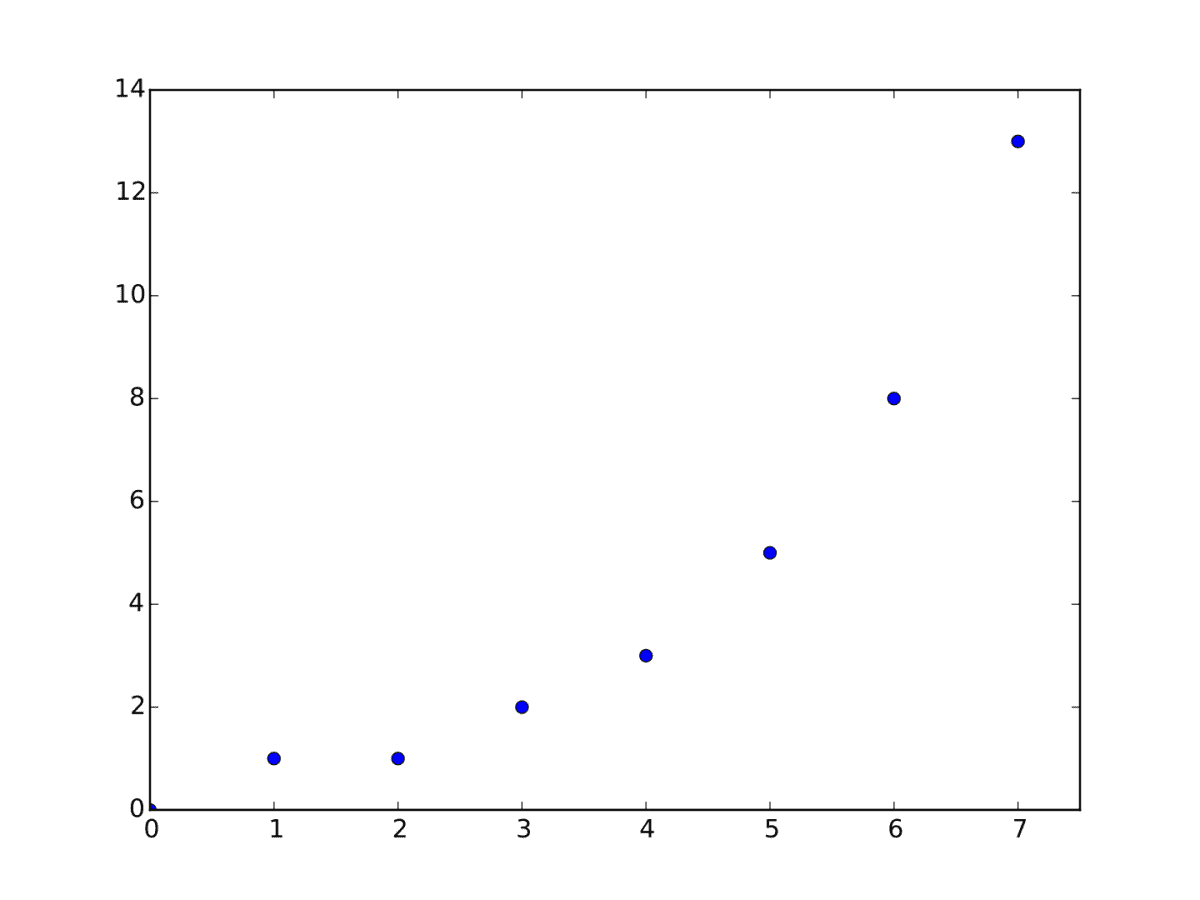The graph of the first several Fibonacci numbers is not linear, which shows us that it is not an arithmetic sequence.

## Conclusion

Now you know what geometric sequences are and what they look like.  You also know the answers to some common questions about geometric sequences.

When you add up the terms of a geometric sequence, you get a geometric series, which you can learn more about in my article here.